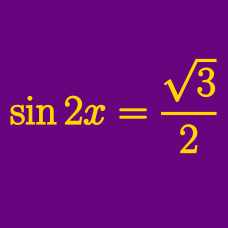Geometry

# General Solution of Simple Trigonometric Equations

Which of the following is the general solution of $2\sqrt{3}=4\sin x?$

Which of the following is the general solution for the equation $\frac{\sqrt{3}}{3}=\tan4x?$

Which of the following is the general solution for the equation $\sqrt{3}=2\sin(12x+\frac{3}{11}\pi)?$

What is the general solution for the equation $8\cos150^\circ=4\tan\left(7x+\frac{3}{7}\pi\right)?$

Which of the following is a general solution of the equation $\tan \left(x+\frac{37}{3}\pi \right) = 1 ?$

×